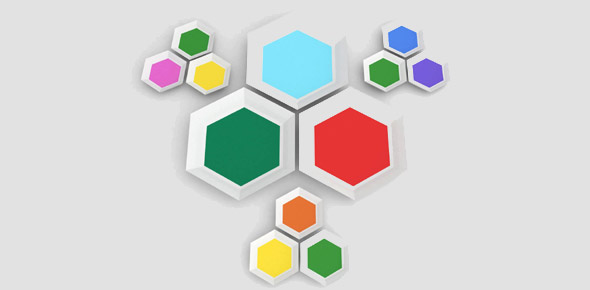# Geometry Shapes And Similarity

12 Questions | Attempts: 171
ShareSettingsA test of geometrical knowledge at Y9 level

• 1.
What has three sides with two the same length?
• A.

A triangle

• B.

An equilateral triangle

• C.

An Isosceles triangle

• 2.
What shape has two pairs of parallel sides with no right-angles?
• A.

A rectangle

• B.

A square

• C.

A parallelogram

• 3.
What shape has 4 lines of symmetry and two pairs of parallel sides?
• A.

A rectangle

• B.

A triangle

• C.

A square

• 4.
Name the shape which has all three angles equal to 60 degrees
• A.

A triangle

• B.

A right triangle

• C.

An equilateral triangle

• 5.
Name a shape with all sides the same length but with only 2 lines of symmetry
• A.

A square

• B.

A rhombus

• C.

A kite

• 6.
What is the name of the shape with four sides but only one pair of parallel sides?
• A.

Parallelogram

• B.

Kite

• C.

Trapezium

• 7.
How many sides has a quadrilateral?
• A.

3

• B.

4

• C.

6

• 8.
How many lines of symmetry does a REGULAR pentagon have?
• A.

3

• B.

5

• C.

2

• 9.
What is the correct name for a shape with six sides all having the same length and with all interior angle equal?
• A.

A Hexagon

• B.

A Regular Hexagon

• C.

An Irregular Hexagon

• 10.
What is the name of a polygon with at least 2 sides the same?
• A.

Isosceles trapezium

• B.

Isosceles triangle

• C.

Regular Triangle

• 11.
How many sides has a dodecagon?
• A.

20

• B.

12

• C.

10

• 12.
What is a tessellation?
• A.

A regular shape

• B.

A collection of the same shapes which cover an area

• C.

Similar shapes which fit together to make a bigger shape

## Related TopicsBack to top
×

Wait!
Here's an interesting quiz for you.